# How to Calculate and Solve for Modulus of Rupture | Fracture Mechanics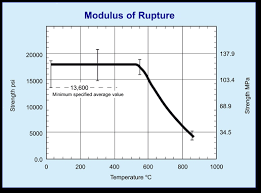The image above represents modulus of rupture.

To compute for modulus of rupture, three essential parameters are needed and these parameters are Bending Moment (M), Distance (c) and Moment of Inertia (I).

The formula for calculating modulus of rapture:

σ = Mc / I

Where:

σ = Modulus of Rupture
M = Bending Moment
c = Distance
I = Moment of Inertia

Let’s solve an example;
Find the modulus of rupture when the bending moment is 22, the distance is 10 and the moment of inertia is 14.

This implies that;

M = Bending Moment = 22
c = Distance = 10
I = Moment of Inertia = 14

σ = Mc / I
σ = 22(10) / 14
σ = 220 / 14
σ = 15.71

Therefore, the modulus of rupture is 15.71 Pa.

Calculating the Bending Moment when the Modulus of Rupture, the Distance and the Moment of Inertia is Given.

M = σ x I / c

Where;

M = Bending Moment
σ = Modulus of Rupture
c = Distance
I = Moment of Inertia

Let’s solve an example;
Find the bending moment when the modulus of rupture is 22, the distance is 8 and the moment of inertia is 2.

This implies that;

σ = Modulus of Rupture = 22
c = Distance = 8
I = Moment of Inertia = 2

M = σ x I / c
M = 22 x 2 / 8
M = 44 / 8
M = 5.5

Therefore, the bending moment is 5.5.

Calculating the Distance when the Modulus of Rupture, the Bending Moment and the Moment of Inertia is Given.

c = σ x I / M

Where;

c = Distance
σ = Modulus of Rupture
M = Bending Moment
I = Moment of Inertia

Let’s solve an example;
Find the distance when the modulus of rupture is 12, the bending moment is 4 and the moment of inertia is 16.

This implies that;

σ = Modulus of Rupture = 12
M = Bending Moment = 4
I = Moment of Inertia = 16

c = σ x I / M
c = 12 x 16 / 4
c = 192 / 4
c = 48

Therefore, the distance is 48.

Calculating the Moment of Inertia when the Modulus of Rupture, the Bending Moment and the Distance is Given.

I = Mc / σ

Where;

I = Moment of Inertia
σ = Modulus of Rupture
M = Bending Moment
c = Distance

Let’s solve an example;
Find the moment of inertia when the modulus of rupture is 30, the bending moment is 10 and the distance is 3.

This implies that;

σ = Modulus of Rupture = 30
M = Bending Moment = 10
c = Distance = 3

I = Mc / σ
I = 10 x 3 / 30
I = 30 / 30
I = 1

Therefore, the moment of inertia is 1.

Nickzom Calculator – The Calculator Encyclopedia is capable of calculating the modulus of rupture.

To get the answer and workings of the modulus of rupture using the Nickzom Calculator – The Calculator Encyclopedia. First, you need to obtain the app.

You can get this app via any of these means:

To get access to the professional version via web, you need to register and subscribe for NGN 2,000 per annum to have utter access to all functionalities.
You can also try the demo version via https://www.nickzom.org/calculator

Android (Paid) – https://play.google.com/store/apps/details?id=org.nickzom.nickzomcalculator
Android (Free) – https://play.google.com/store/apps/details?id=com.nickzom.nickzomcalculator
Apple (Paid) – https://itunes.apple.com/us/app/nickzom-calculator/id1331162702?mt=8
Once, you have obtained the calculator encyclopedia app, proceed to the Calculator Map, then click on Materials and Metallurgical under Engineering.Now, Click on Fracture Mechanics under Materials and MetallurgicalNow, Click on Modulus of Rupture under Fracture Mechanics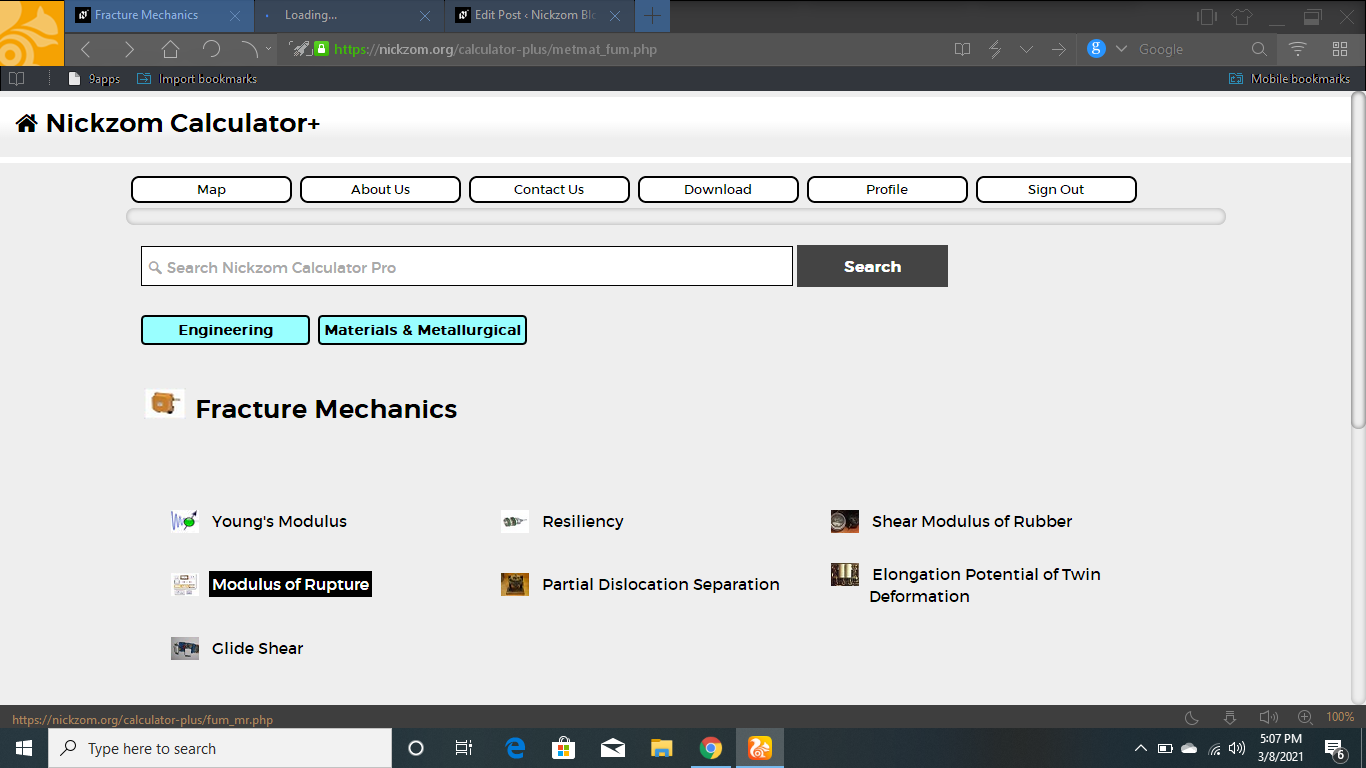The screenshot below displays the page or activity to enter your values, to get the answer for the modulus of rupture according to the respective parameters which is the Bending Moment (M), Distance (c) and Moment of Inertia (I).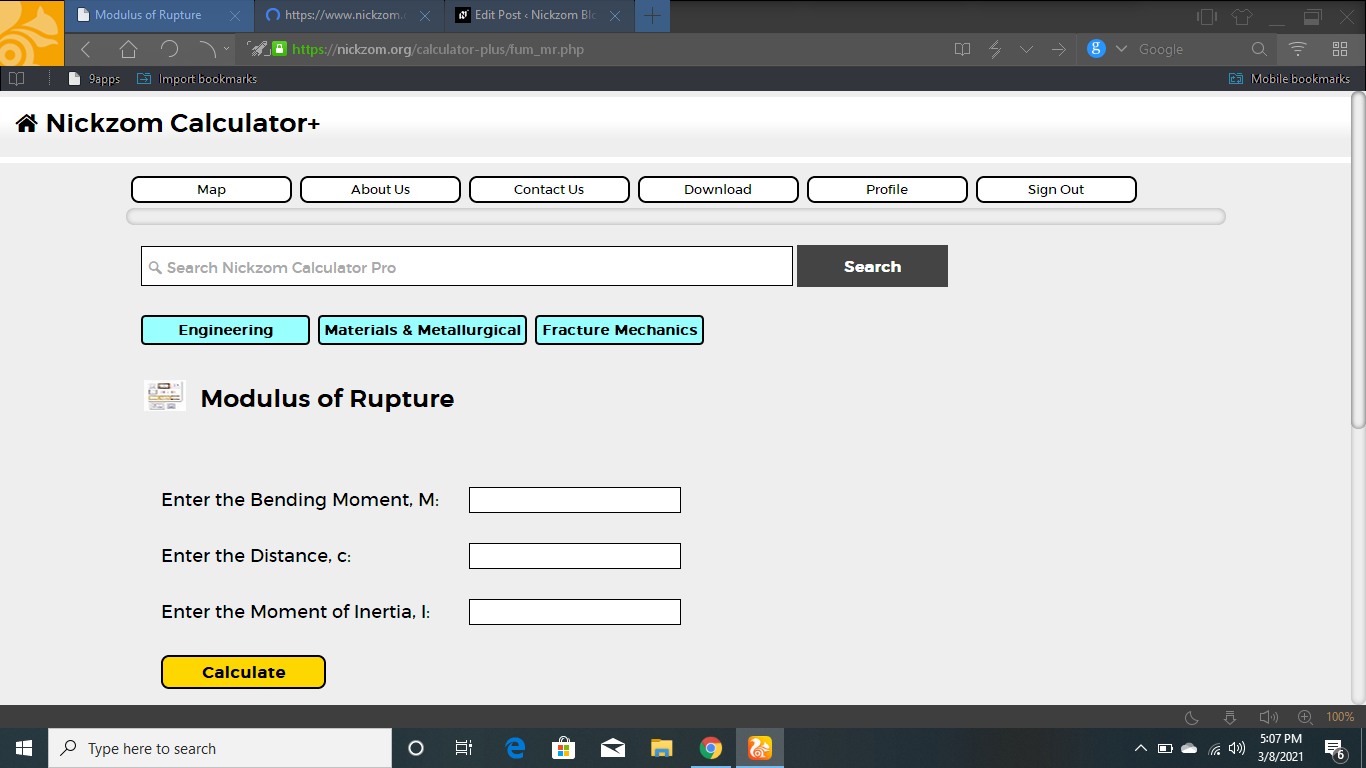Now, enter the value appropriately and accordingly for the parameter as required by the Bending Moment (M) is 22, Distance (c) is 10 and Moment of Inertia (I) is 14.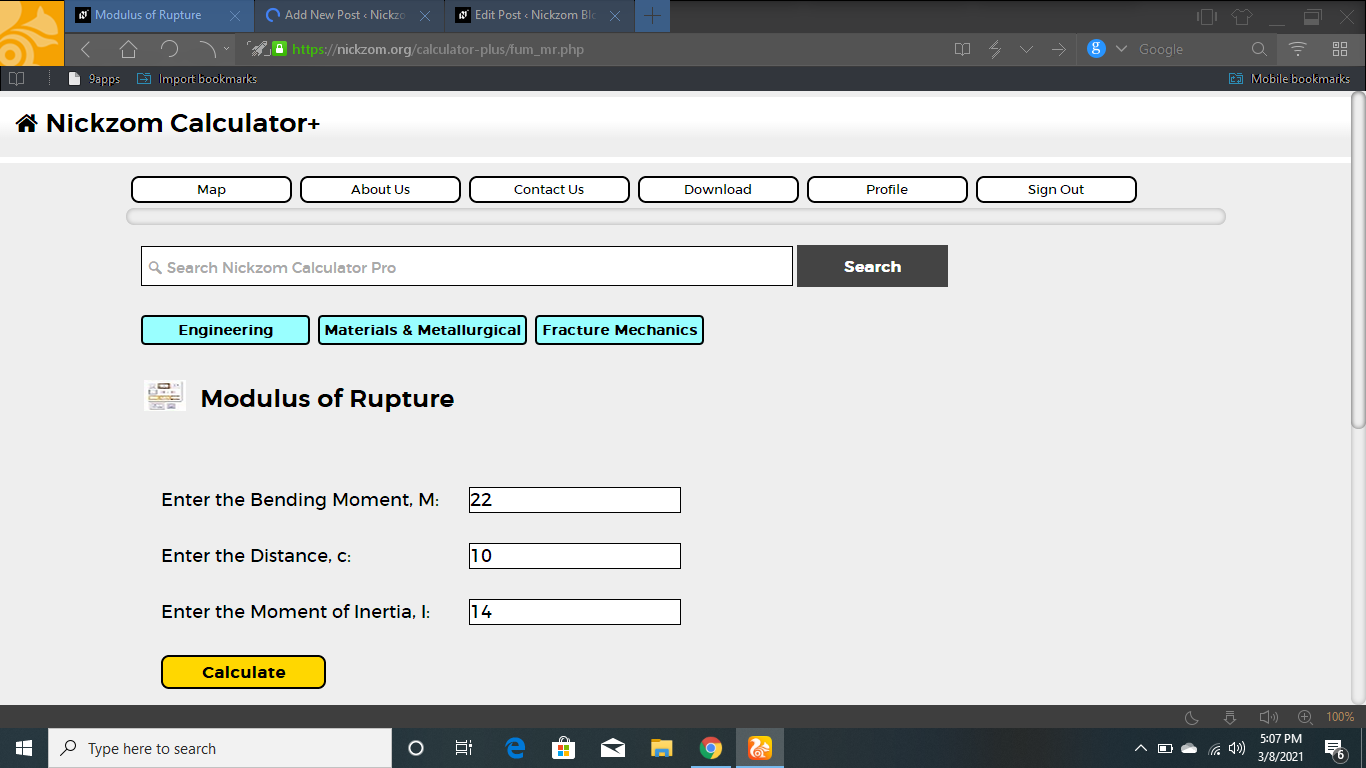Finally, Click on Calculate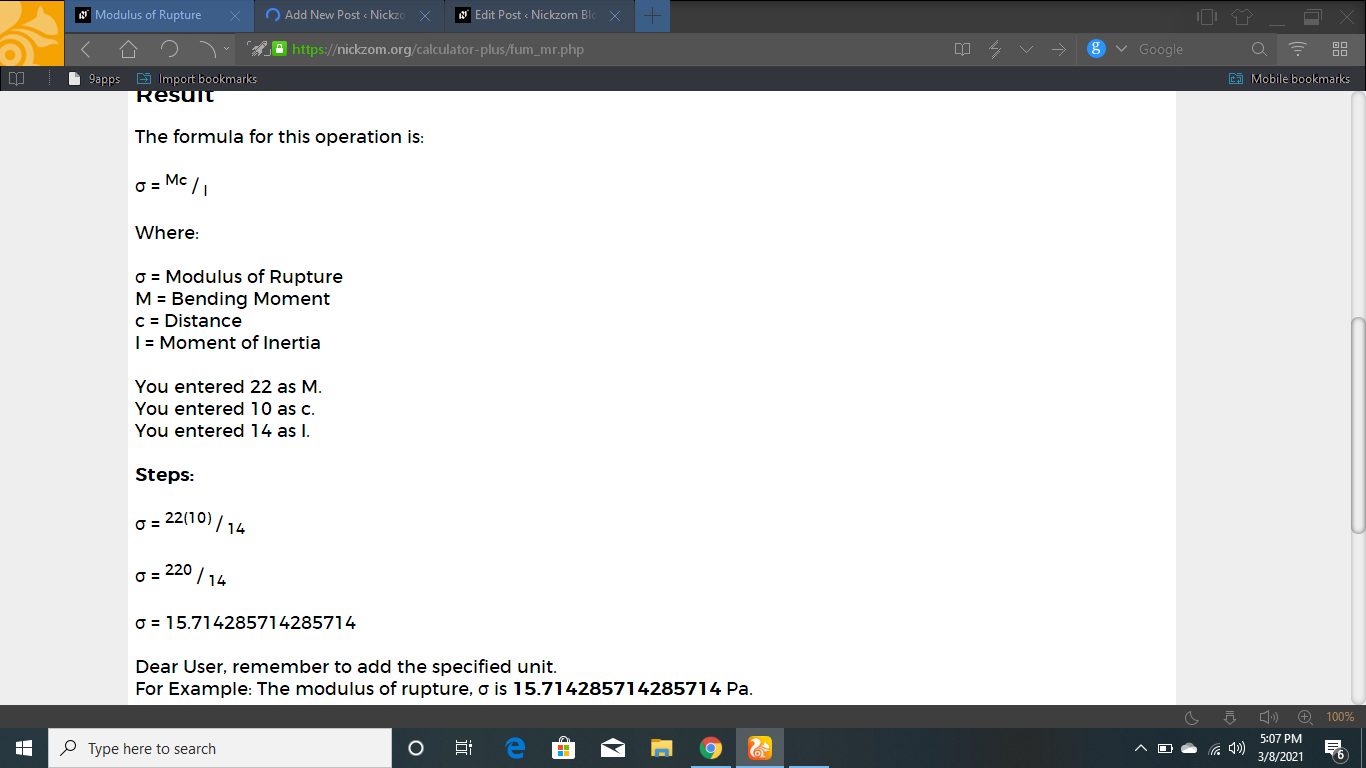As you can see from the screenshot above, Nickzom Calculator– The Calculator Encyclopedia solves for the modulus of rupture and presents the formula, workings and steps too.